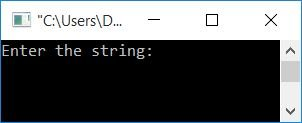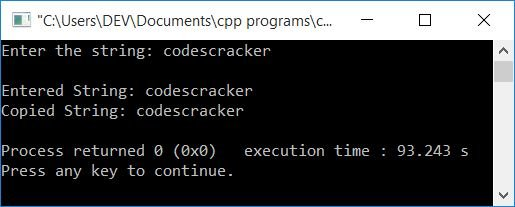# C++ Program to Copy One String to Another

In this article, you will learn how to copy one string to another in C++ and get the code for it. The program is created using the following approaches:

## Copy a string without using the strcpy() function in C++

This program copies the string (entered by the user at run-time) without using any library function like strcpy(). Let's have a look at the program first. Its explanation is given later on.

The question is, "Write a program in C++ that copies one string to another." Here is its answer:

```#include<iostream>
#include<stdio.h>
using namespace std;
int main()
{
char strOrig, strCopy, i=0;
cout<<"Enter the string: ";
gets(strOrig);
while(strOrig[i]!='\0')
{
strCopy[i] = strOrig[i];
i++;
}
strCopy[i] = '\0';
cout<<"\nEntered String: "<<strOrig;
cout<<"\nCopied String: "<<strCopy;
cout<<endl;
return 0;
}```

This program was built and runs under the Code::Blocks IDE. Here is its sample run:Now enter the string, say "codescracker," and press the ENTER key to copy it to another string. Here is the final snapshot of its sample run:The dry run of the above program with user input of "codescracker" goes like this:

• Initial value, i=0
• When the user enters the string, say "codescracker," it gets stored in a variable, strOrig[], in the following way:
• strOrig=c
• strOrig=o
• strOrig=d
• strOrig=e
• strOrig=s
• strOrig=c
• strOrig=r
• strOrig=a
• strOrig=c
• strOrig=k
• strOrig=e
• strOrig=r
• That is, the first character (c) of the string (codescracker) is stored at the strOrig['s] very first index (0). And the second character (o) gets stored at the second index (1), and so on.
• Because the maximum size of strOrig[] is 100, and the entered string is only 12 characters long, at the 12th index (the index after the last character's index), a null-terminated character (\0) gets automatically initialized. That indicates the end of the string, or that there are no more characters in strOrig from the 12th index.
• Now the condition, strOrig[i]!='\0' or strOrig!='\0' or c!='\0', evaluates to be true, therefore program flow goes inside the loop and the 0th index's character of strOrig[] gets initialized to strCopy[] at the same index.
• That is, strOrig[i] or strOrig or c gets initialized to strCopy[i] or strCopy.
• The value of i is now incremented. So i=1
• Program flow goes back and evaluates the condition of the while loop again.
• Because a null-terminated character (\0) is available at the 12th index, both the statements (available in the body of the while loop) gets executed 12 times.
• As a result, each character is copied to the strCopy[] variable one by one.
• When the while loop is finished, don't forgot to initialize a null-terminated character at the index following the last character's index.
• Simply print the values of both variables, strOrig[] and strCopy[].

## Copying strings in C++ using a pointer

This program copies the string using a pointer. The question is: write a program in C++ to copy the content of one string to another using a pointer. Here is its answer:

```#include<iostream>
#include<stdio.h>
using namespace std;
int main()
{
char strOrig, strCopy;
char *origPtr, *copPtr;
cout<<"Enter the string: ";
gets(strOrig);
origPtr = &strOrig;
copPtr = &strCopy;
while(*origPtr)
{
*copPtr = *origPtr;
origPtr++;
copPtr++;
}
*copPtr = '\0';
cout<<"\nEntered String: "<<strOrig;
cout<<"\nCopied String: "<<strCopy;
cout<<endl;
return 0;
}```

Here is its sample run with user input: Hello codescrackerThe statement,

`origPtr = &strOrig;`

initializes the strOrig[] variable's initial address. That is its 0th index's address. And the statement,

`copPtr = &strCopy;`

also sets the address of strCopy[] to the 0th.

Note: If a pointer-type variable holds the address of any variable that contains some values, then anything that happens with the variable (pointer) makes changes to the original. because it holds its address, not value. So any operation performed through a pointer-type variable directly effects the value at that address.

The * is called as the value at operator. And & is called as the address of operator.

The statement, `origPtr++;`, means that origPtr now holds the next index's address.

So, using the pointer, all of the characters from the original string are copied (one by one) to strCopy[].

## Copy a string in C++ using a user-defined function

This program also copies one string to another, but using a user-defined function named cpystr().

```#include<iostream>
#include<stdio.h>
using namespace std;
void cpystr(char *, char *);
int main()
{
char strOrig, strCopy;
cout<<"Enter the string: ";
gets(strOrig);
cpystr(strOrig, strCopy);
cout<<"\nEntered String: "<<strOrig;
cout<<"\nCopied String: "<<strCopy;
cout<<endl;
return 0;
}
void cpystr(char *origPtr, char *copPtr)
{
while(*origPtr)
{
*copPtr = *origPtr;
origPtr++;
copPtr++;
}
*copPtr = '\0';
}```

This program produces the same output as the previous program.

### Copy a string in C++ using the strcpy() function

This is the last program that uses a library function of C++ named strcpy() to copy a string. It takes two strings as its argument. The second argument's value is copied to the first.

```#include<iostream>
#include<stdio.h>
#include<string.h>
using namespace std;
int main()
{
char strOrig, strCopy;
cout<<"Enter the string: ";
gets(strOrig);
strcpy(strCopy, strOrig);
cout<<"\nEntered String: "<<strOrig;
cout<<"\nCopied String: "<<strCopy;
cout<<endl;
return 0;
}```

#### The same program in different languages

C++ Quiz

« Previous Program Next Program »